# Demand Paged Virtual Memory Mark Stanovich Operating Systems

• Slides: 92Demand Paged Virtual Memory Mark Stanovich Operating Systems COP 4610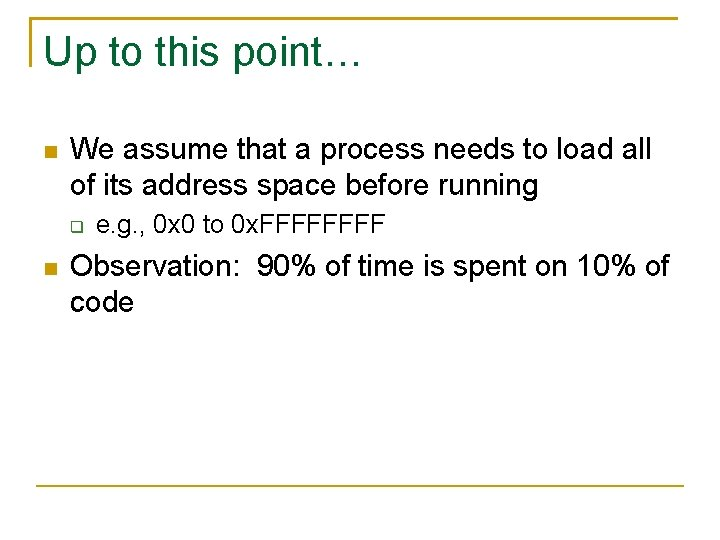Up to this point… We assume that a process needs to load all of its address space before running e. g. , 0 x 0 to 0 x. FFFF Observation: 90% of time is spent on 10% of codeDemand Paging Demand paging: allows pages that are referenced actively to be loaded into memory Remaining pages stay on disk Provides the illusion of infinite physical memory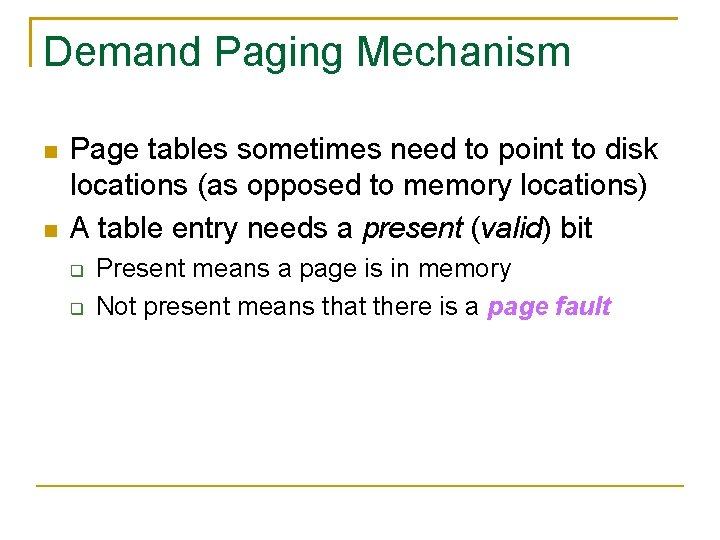Demand Paging Mechanism Page tables sometimes need to point to disk locations (as opposed to memory locations) A table entry needs a present (valid) bit Present means a page is in memory Not present means that there is a page faultPage Fault Hardware trap OS performs the following steps while running other processes (analogy: firing and hiring someone) Choose a page If the page has been modified, write its contents to disk Change the corresponding page table entry and TLB entry Load new page into memory from disk Update page table entry Continue thread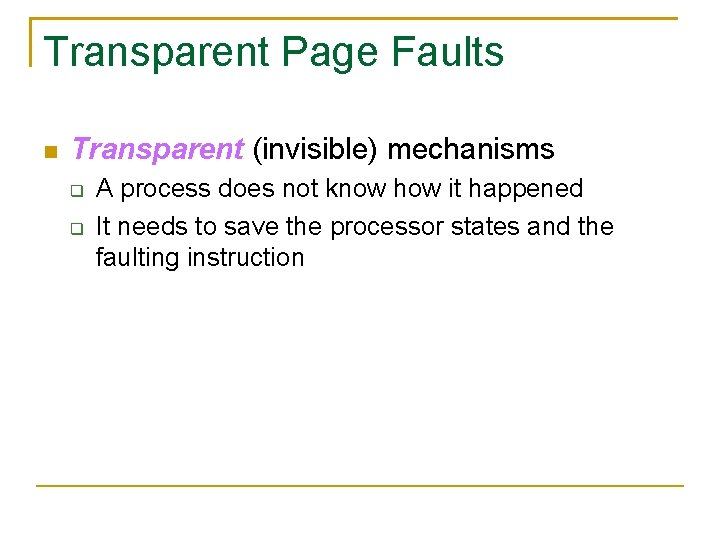Transparent Page Faults Transparent (invisible) mechanisms A process does not know how it happened It needs to save the processor states and the faulting instruction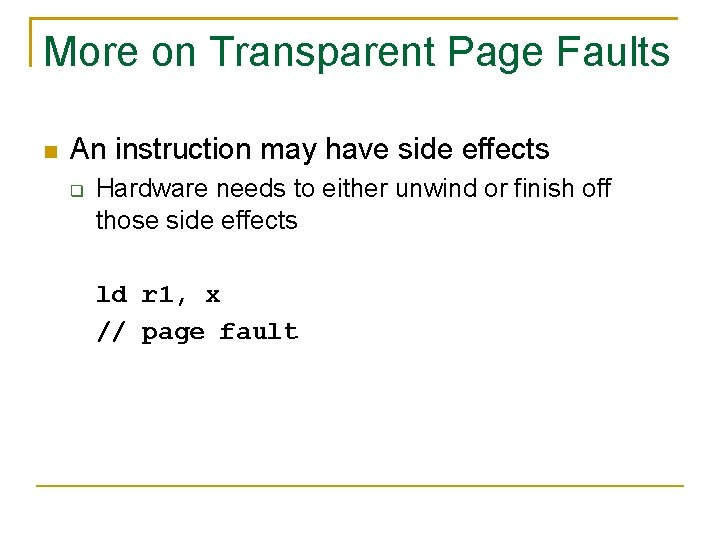More on Transparent Page Faults An instruction may have side effects Hardware needs to either unwind or finish off those side effects ld r 1, x // page faultMore on Transparent Page Faults Hardware designers need to understand virtual memory Unwinding instructions not always possible Example: block transfer instruction source begin block trans dest begin dest end source endPage Replacement Policies Random replacement: replace a random page + Easy to implement in hardware (e. g. , TLB) - May toss out useful pages First in, first out (FIFO): toss out the oldest page + Fair for all pages - May toss out pages that are heavily used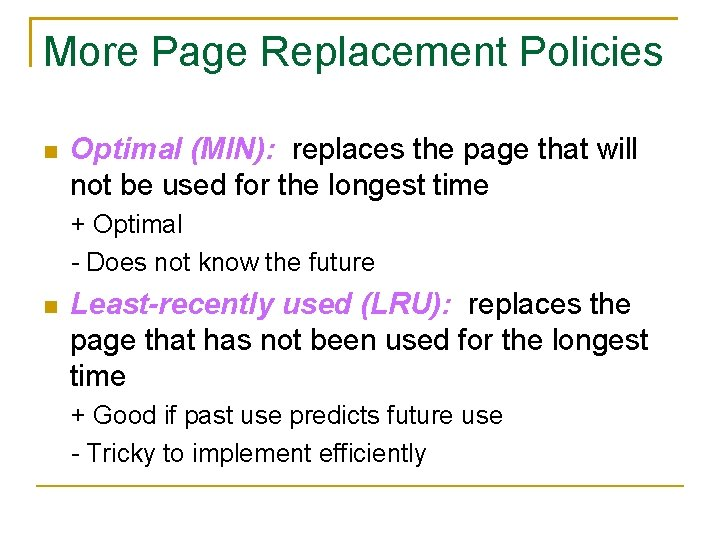More Page Replacement Policies Optimal (MIN): replaces the page that will not be used for the longest time + Optimal - Does not know the future Least-recently used (LRU): replaces the page that has not been used for the longest time + Good if past use predicts future use - Tricky to implement efficientlyMore Page Replacement Policies Least frequently used (LFU): replaces the page that is used least often Tracks usage count of pages + Good if past use predicts future use - Difficult to replace pages with high countsExample A process makes references to 4 pages: A, B, E, and R Reference stream: BEERBAREBEAR Physical memory size: 3 pages Beer?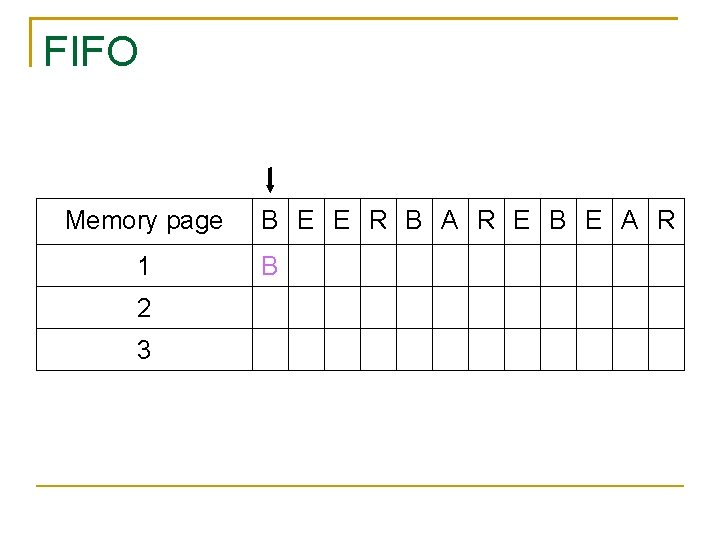FIFO Memory page 1 2 3 B E E R B A R E B E A R B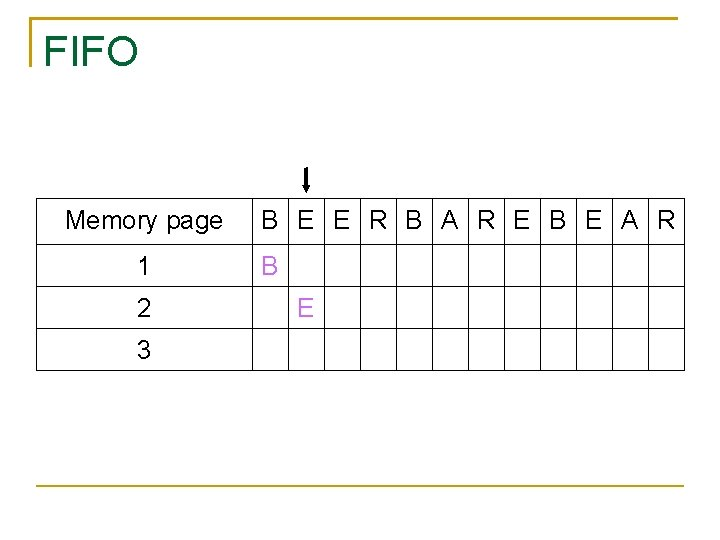FIFO Memory page 1 2 3 B E E R B A R E B E A R B EFIFO Memory page 1 2 3 B E E R B A R E B E A R B E *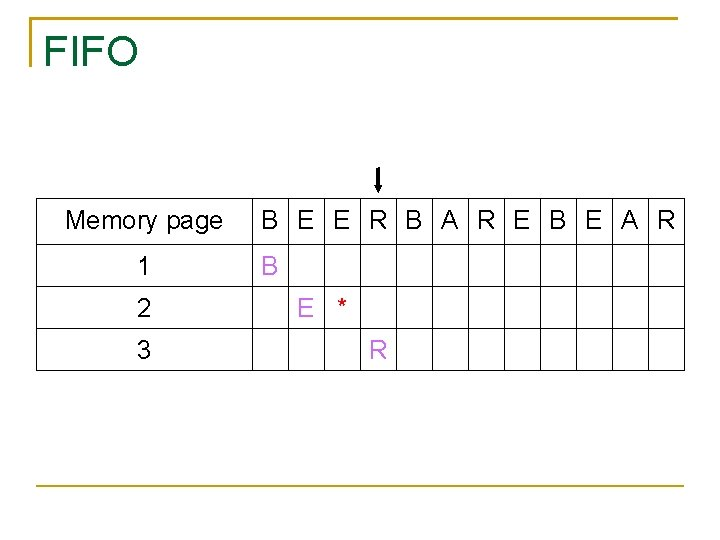FIFO Memory page 1 2 3 B E E R B A R E B E A R B E * RFIFO Memory page 1 2 3 B E E R B A R E B E A R B * E * RFIFO Memory page 1 2 3 B E E R B A R E B E A R B * E * R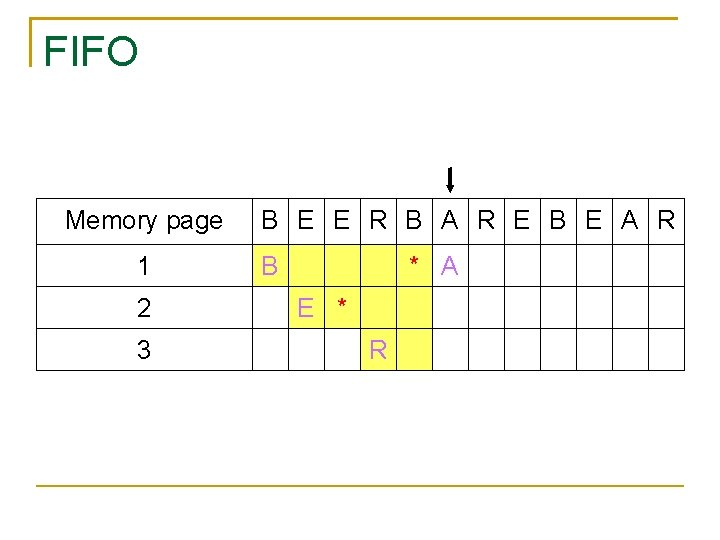FIFO Memory page 1 2 3 B E E R B A R E B E A R B * A E * R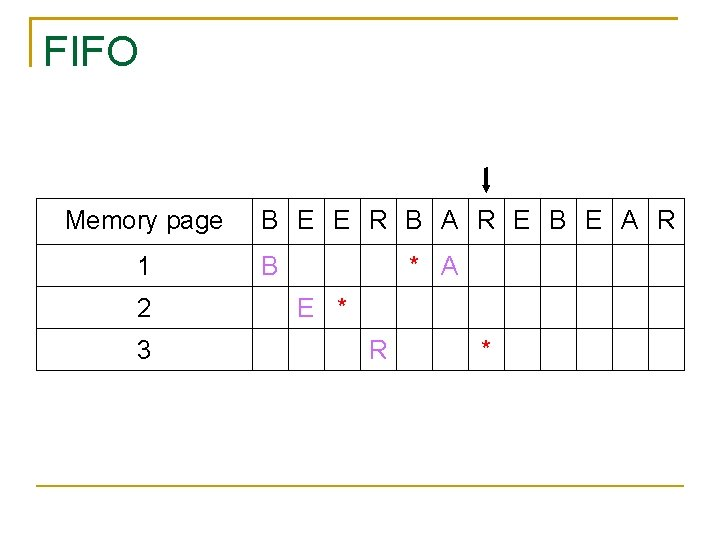FIFO Memory page 1 2 3 B E E R B A R E B E A R B * A E * R *FIFO Memory page 1 2 3 B E E R B A R E B E A R B * A E * * R *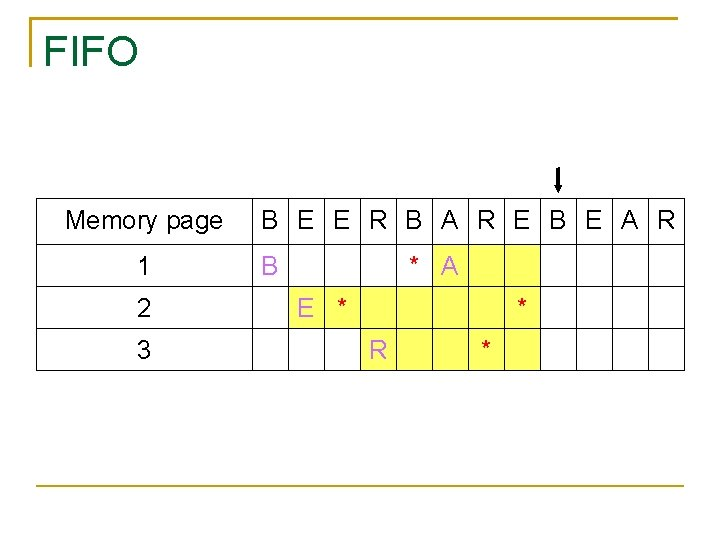FIFO Memory page 1 2 3 B E E R B A R E B E A R B * A E * * R *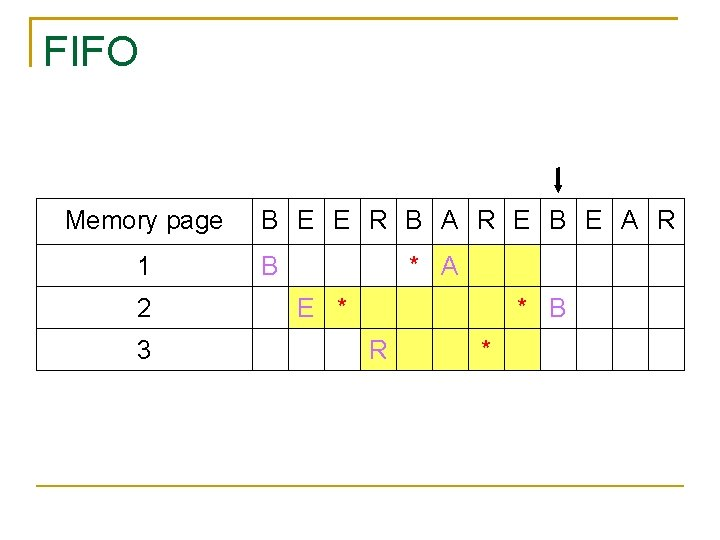FIFO Memory page 1 2 3 B E E R B A R E B E A R B * A E * * B R *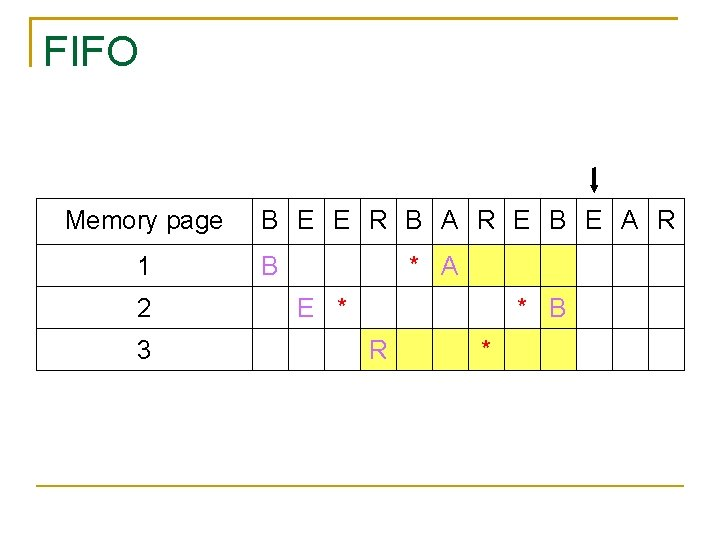FIFO Memory page 1 2 3 B E E R B A R E B E A R B * A E * * B R *FIFO Memory page 1 2 3 B E E R B A R E B E A R B * A E * * B R * E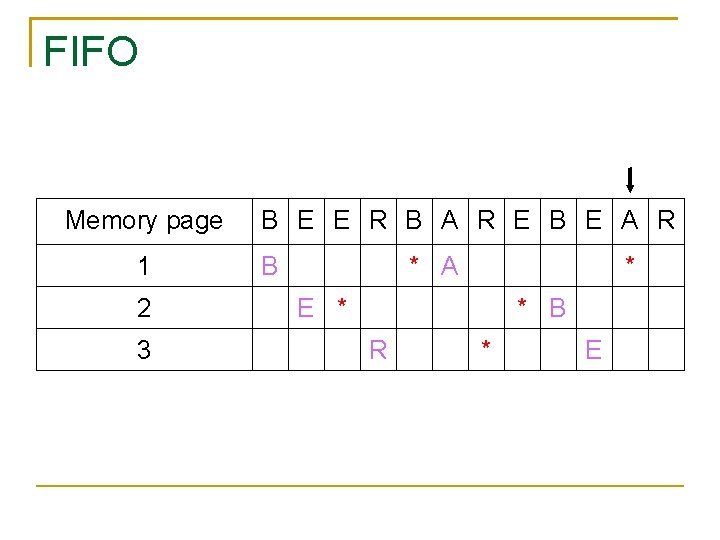FIFO Memory page 1 2 3 B E E R B A R E B E A R B * A * E * * B R * E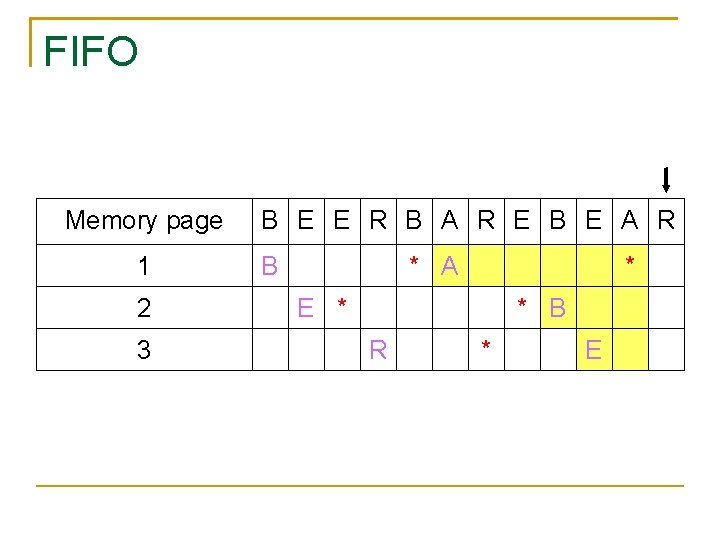FIFO Memory page 1 2 3 B E E R B A R E B E A R B * A * E * * B R * EFIFO Memory page 1 2 3 B E E R B A R E B E A R B * A * R E * * B R * E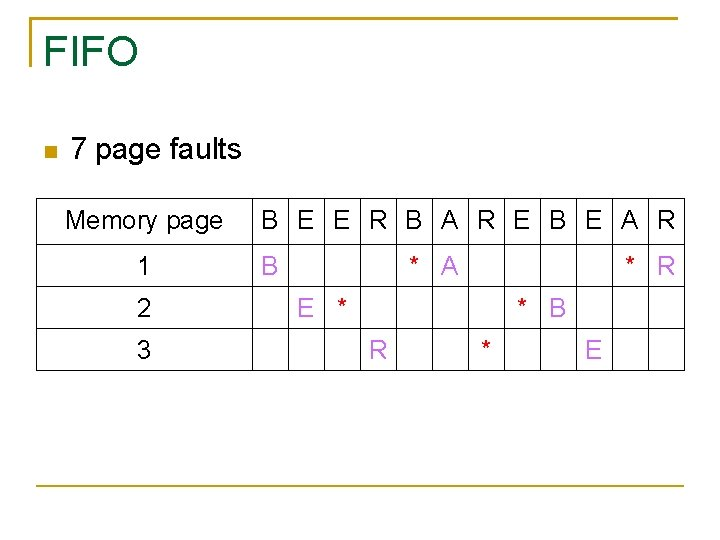FIFO 7 page faults Memory page 1 2 3 B E E R B A R E B E A R B * A * R E * * B R * EFIFO 4 compulsory cache misses Memory page 1 2 3 B E E R B A R E B E A R B * A * R E * * B R * E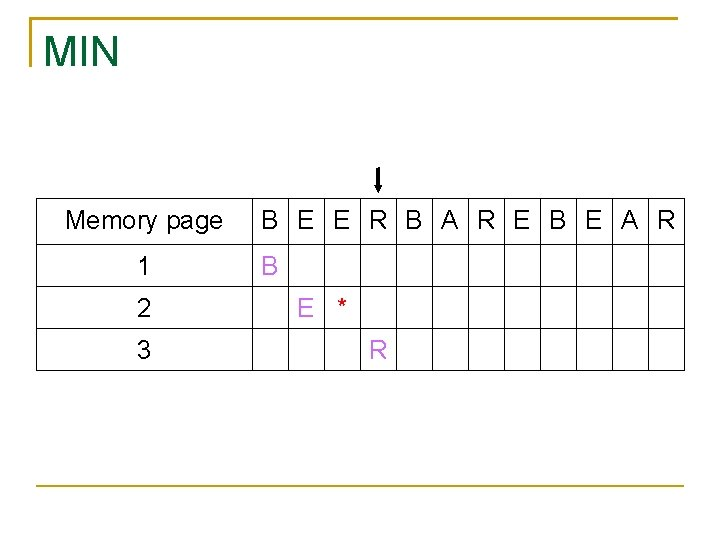MIN Memory page 1 2 3 B E E R B A R E B E A R B E * RMIN Memory page 1 2 3 B E E R B A R E B E A R B * E * R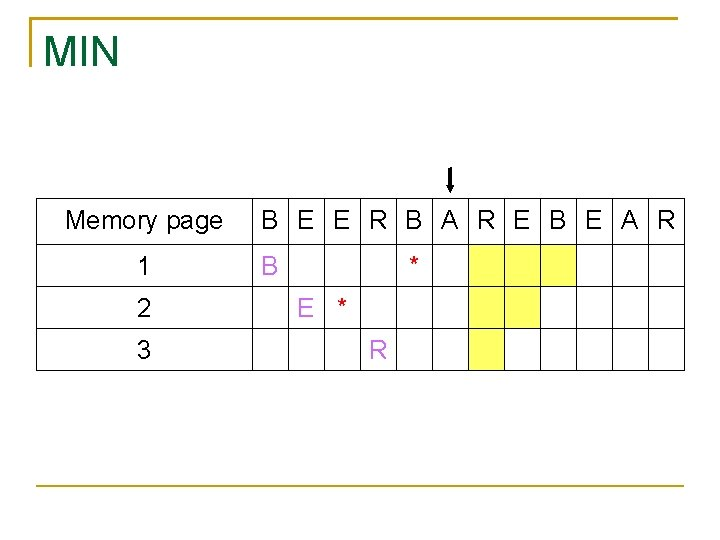MIN Memory page 1 2 3 B E E R B A R E B E A R B * E * RMIN Memory page 1 2 3 B E E R B A R E B E A R B * A E * R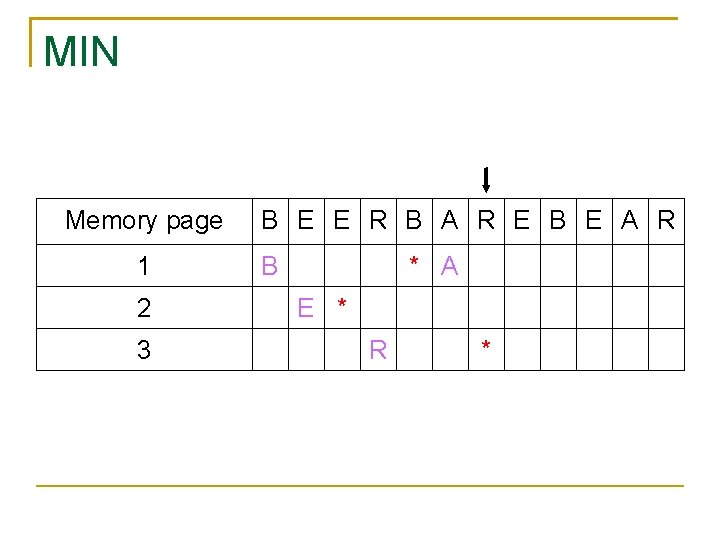MIN Memory page 1 2 3 B E E R B A R E B E A R B * A E * R *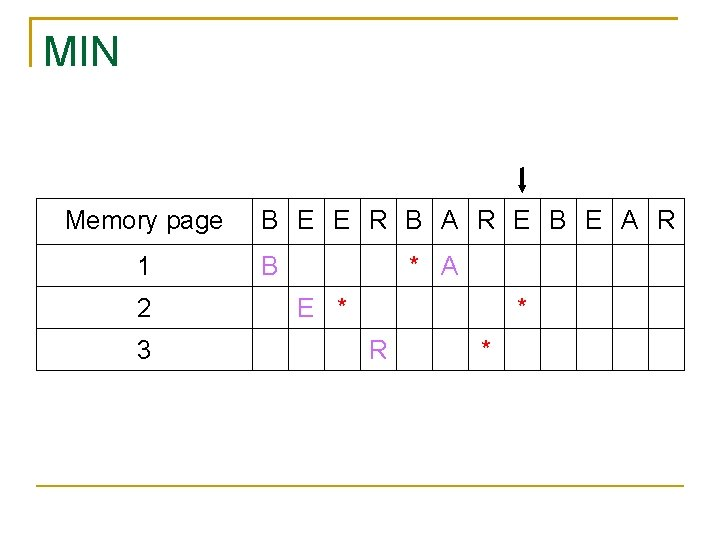MIN Memory page 1 2 3 B E E R B A R E B E A R B * A E * * R *MIN Memory page 1 2 3 B E E R B A R E B E A R B * A E * * R *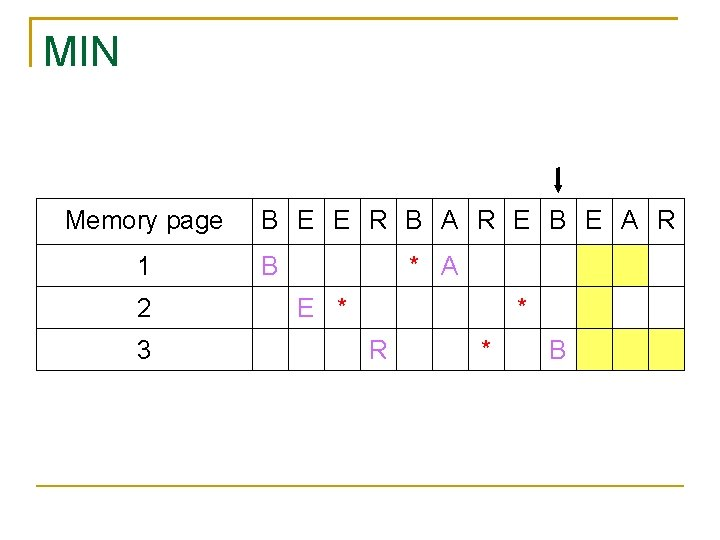MIN Memory page 1 2 3 B E E R B A R E B E A R B * A E * * R * B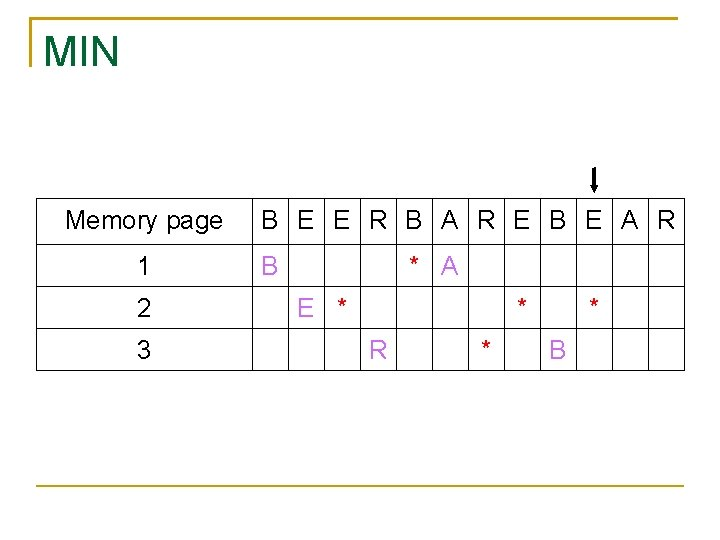MIN Memory page 1 2 3 B E E R B A R E B E A R B * A E * * R * * BMIN Memory page 1 2 3 B E E R B A R E B E A R B * A * E * * R * * B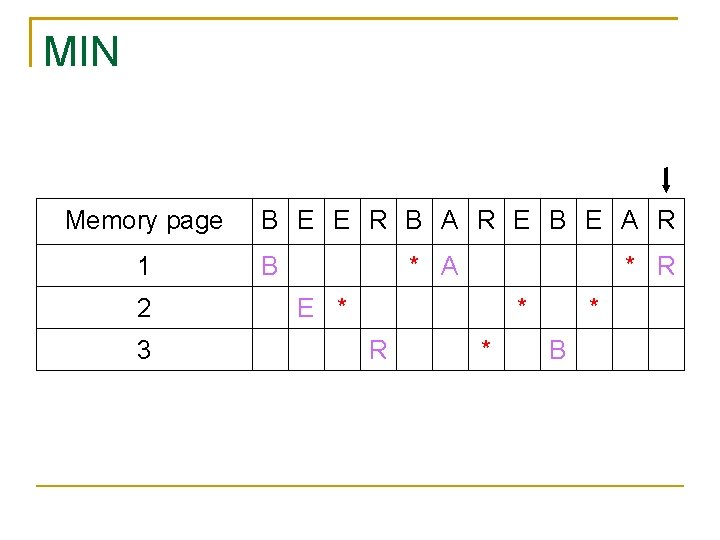MIN Memory page 1 2 3 B E E R B A R E B E A R B * A * R E * * R * * B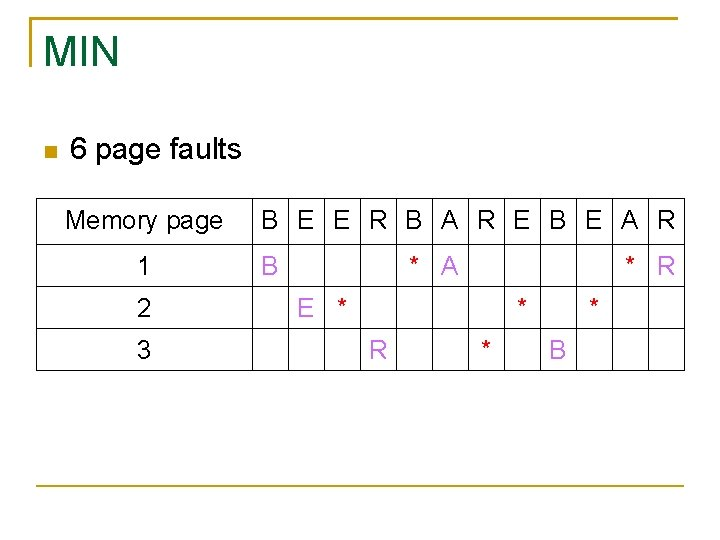MIN 6 page faults Memory page 1 2 3 B E E R B A R E B E A R B * A * R E * * R * * B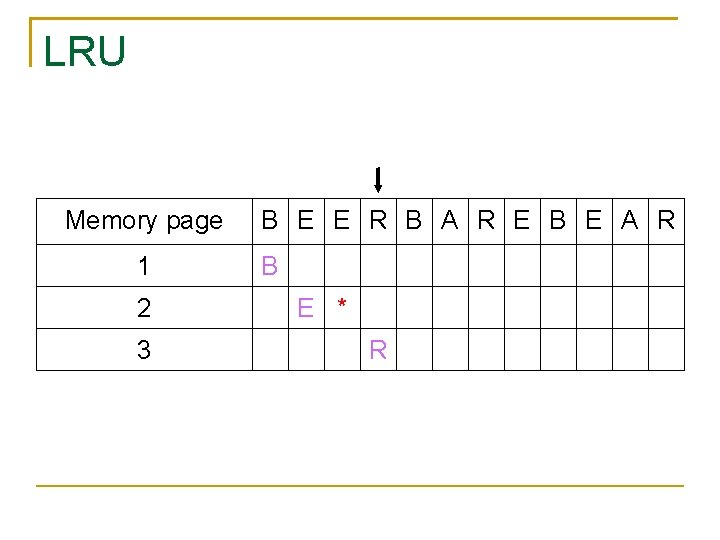LRU Memory page 1 2 3 B E E R B A R E B E A R B E * RLRU Memory page 1 2 3 B E E R B A R E B E A R B * E * R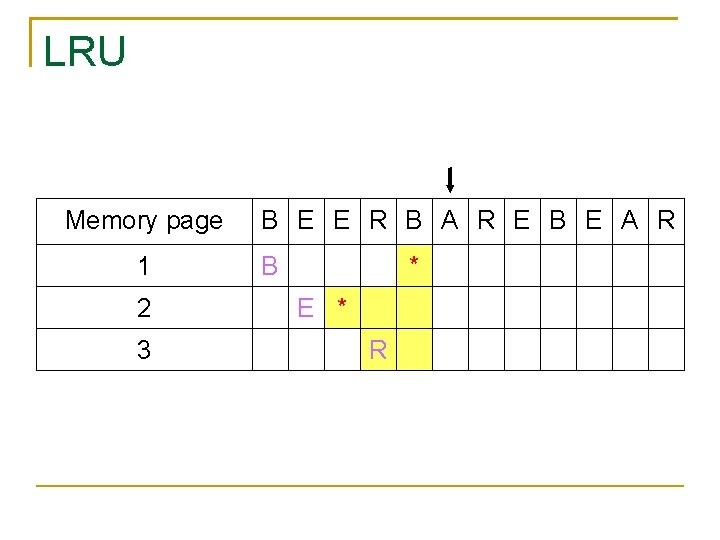LRU Memory page 1 2 3 B E E R B A R E B E A R B * E * R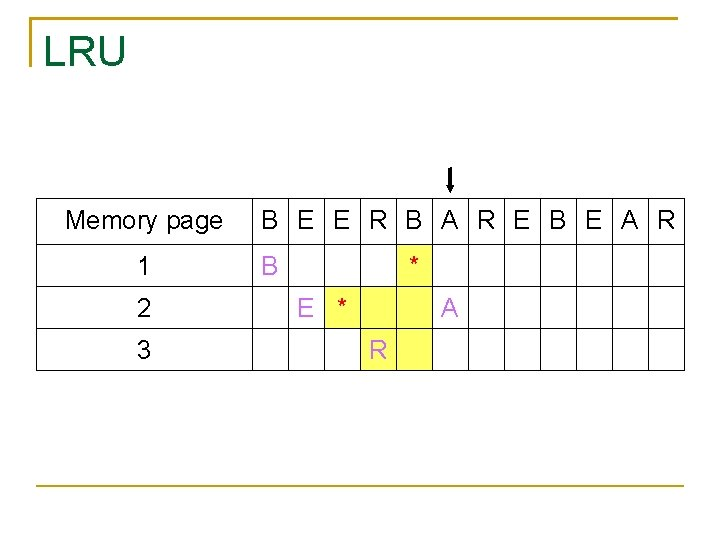LRU Memory page 1 2 3 B E E R B A R E B E A R B * E * A R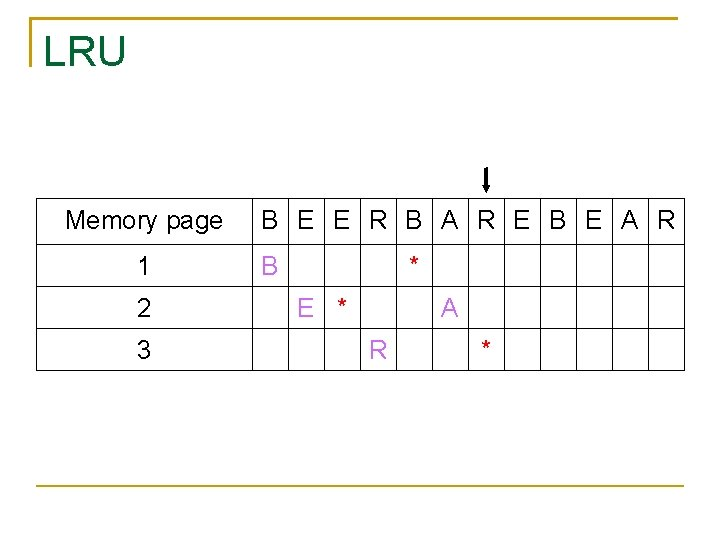LRU Memory page 1 2 3 B E E R B A R E B E A R B * E * A R *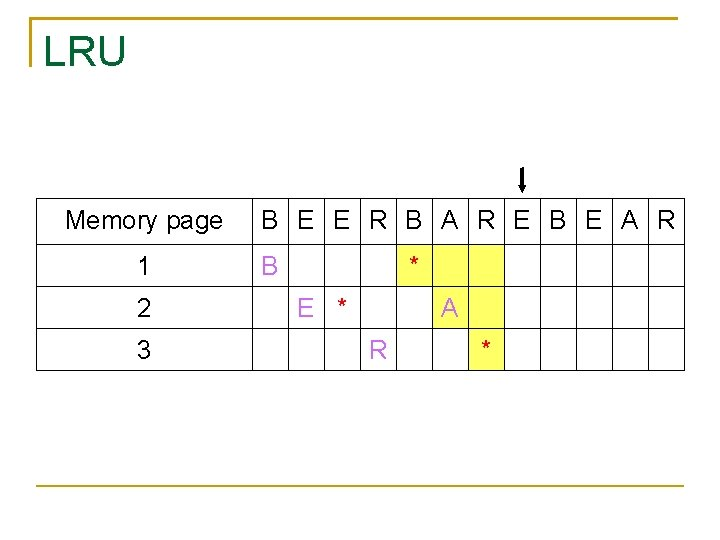LRU Memory page 1 2 3 B E E R B A R E B E A R B * E * A R *LRU Memory page 1 2 3 B E E R B A R E B E A R B * E A R *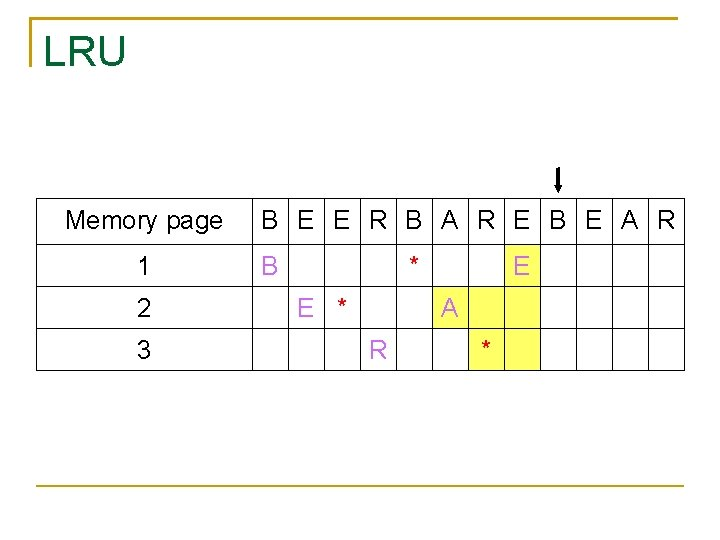LRU Memory page 1 2 3 B E E R B A R E B E A R B * E A R *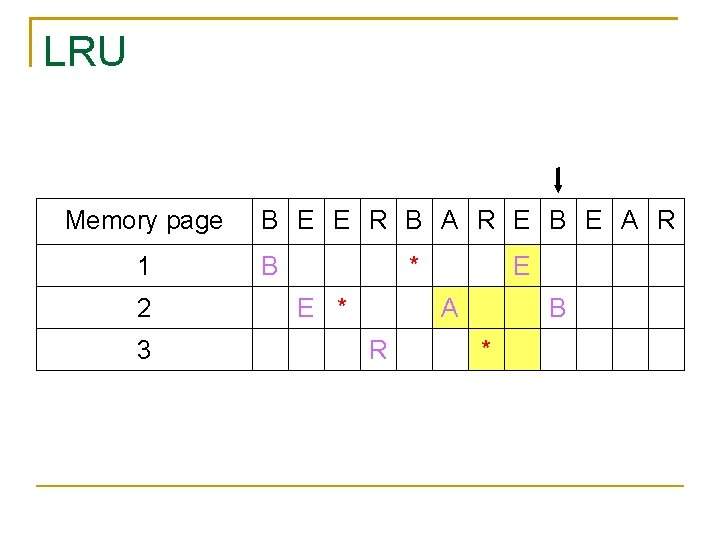LRU Memory page 1 2 3 B E E R B A R E B E A R B *LRU Memory page 1 2 3 B E E R B A R E B E A R B * E A R * B *LRU Memory page 1 2 3 B E E R B A R E B E A R B * E A R * B *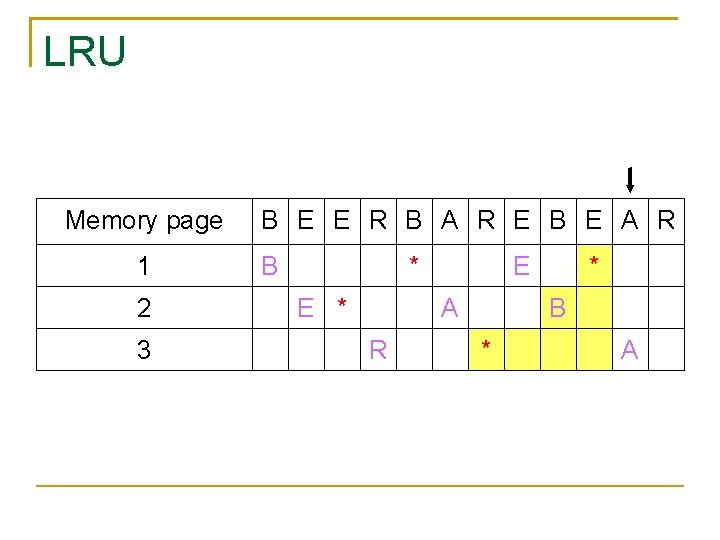LRU Memory page 1 2 3 B E E R B A R E B E A R B * E A R * B * ALRU Memory page 1 2 3 B E E R B A R E B E A R B * E A R * B * A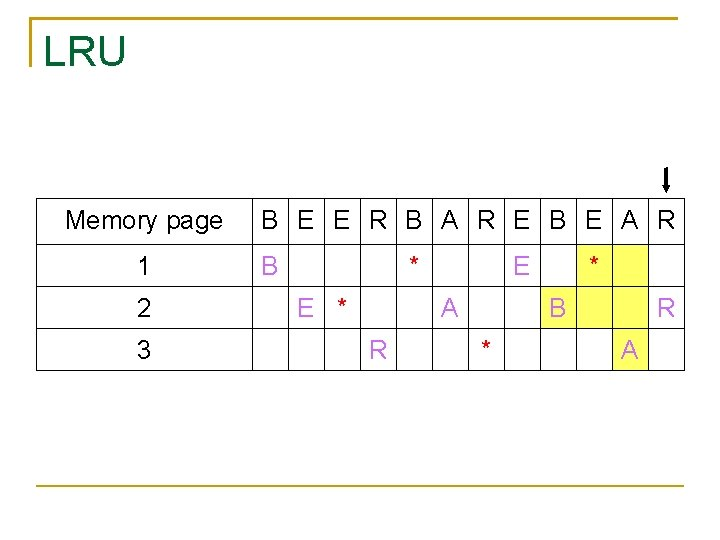LRU Memory page 1 2 3 B E E R B A R E B E A R B * E A R * B * R A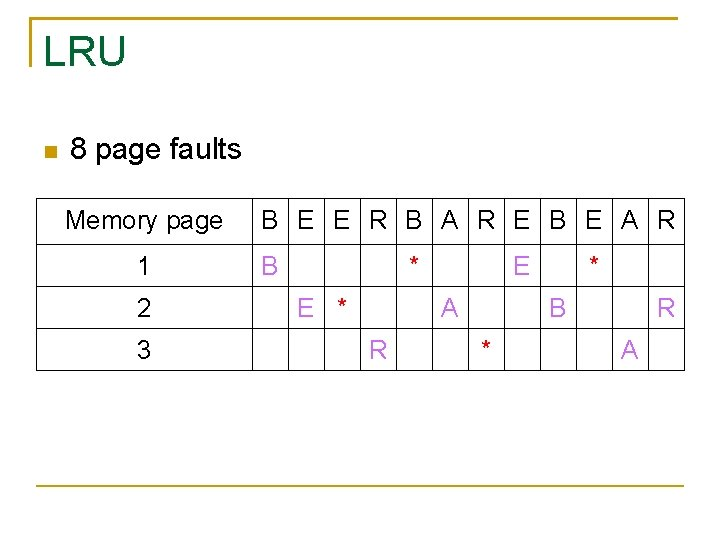LRU 8 page faults Memory page 1 2 3 B E E R B A R E B E A R B * E A R * B * R ALFU Memory page 1 2 3 B E E R B A R E B E A R BLFU Memory page 1 2 3 B E E R B A R E B E A R B E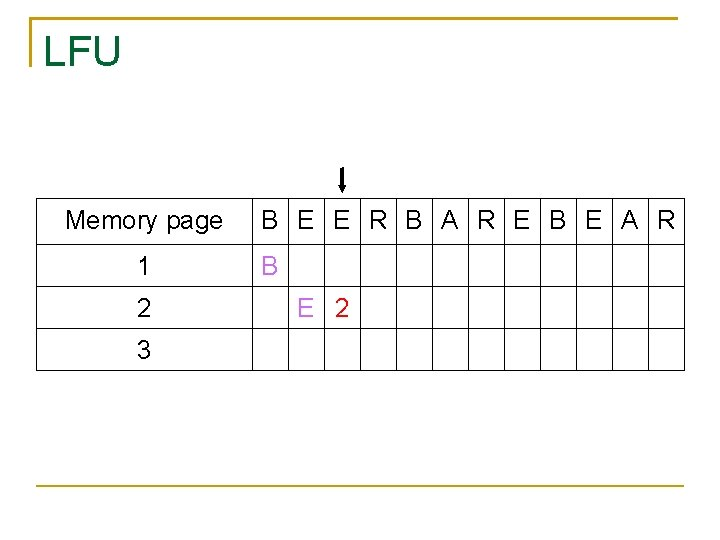LFU Memory page 1 2 3 B E E R B A R E B E A R B E 2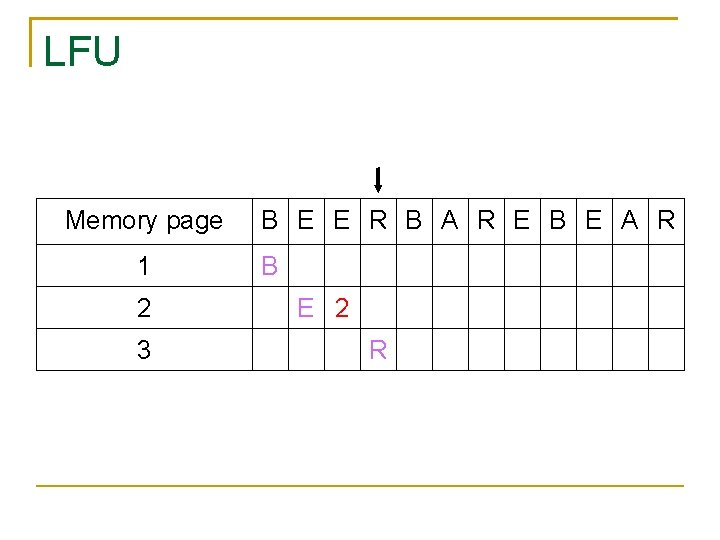LFU Memory page 1 2 3 B E E R B A R E B E A R B E 2 R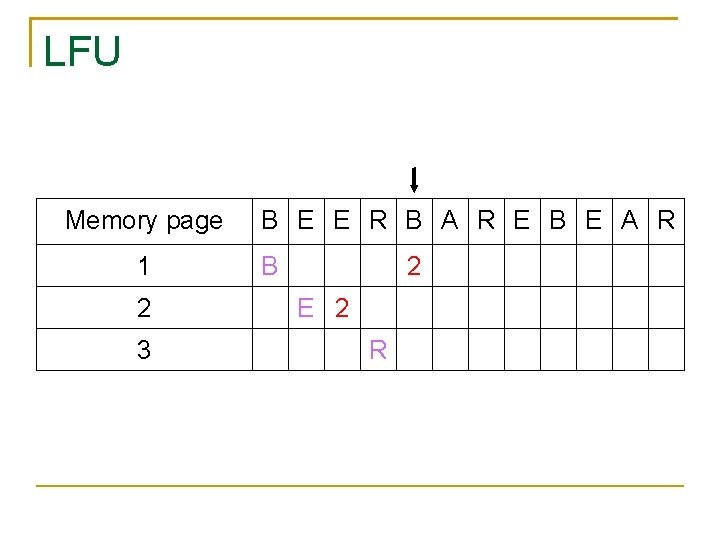LFU Memory page 1 2 3 B E E R B A R E B E A R B 2 E 2 R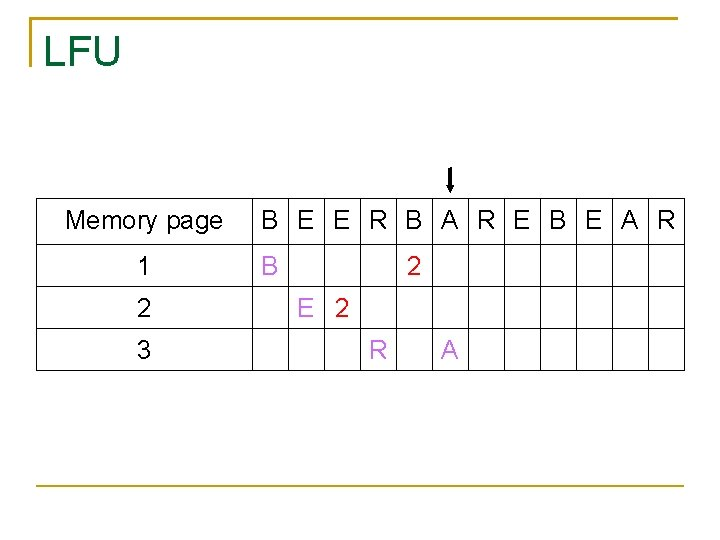LFU Memory page 1 2 3 B E E R B A R E B E A R B 2 E 2 R A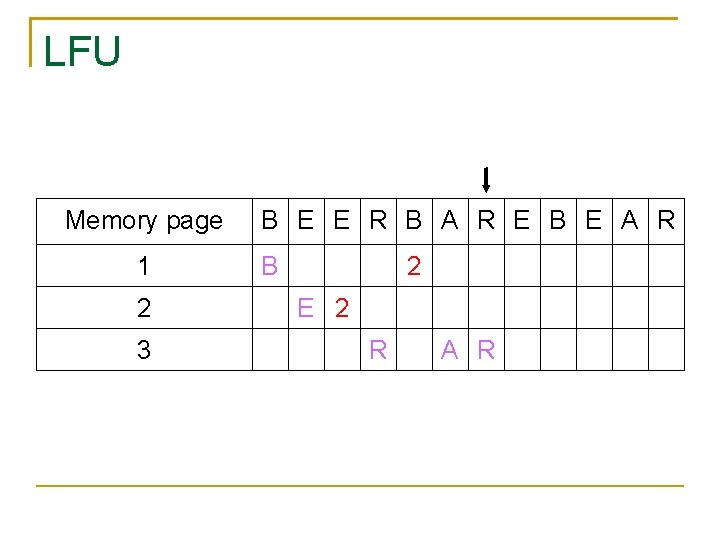LFU Memory page 1 2 3 B E E R B A R E B E A R B 2 E 2 R A RLFU Memory page 1 2 3 B E E R B A R E B E A R B 2 E 2 3 R A R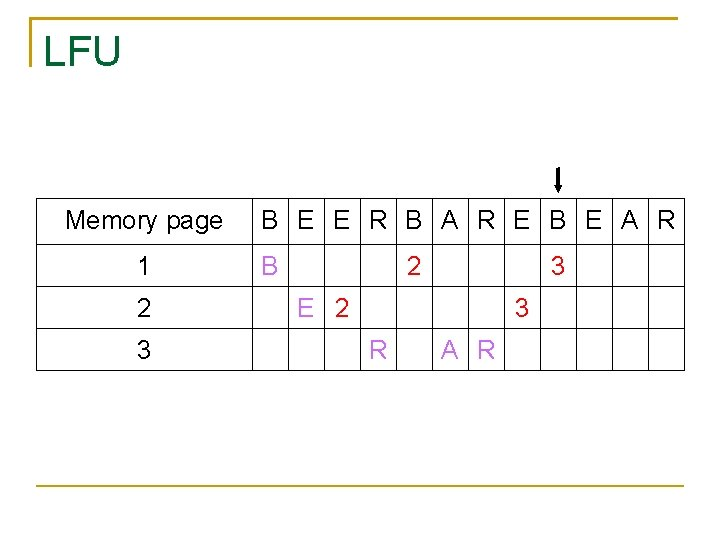LFU Memory page 1 2 3 B E E R B A R E B E A R B 2 3 E 2 3 R A RLFU Memory page 1 2 3 B E E R B A R E B E A R B 2 3 E 2 3 R A R 4LFU Memory page 1 2 3 B E E R B A R E B E A R B 2 3 E 2 3 R A R 4 A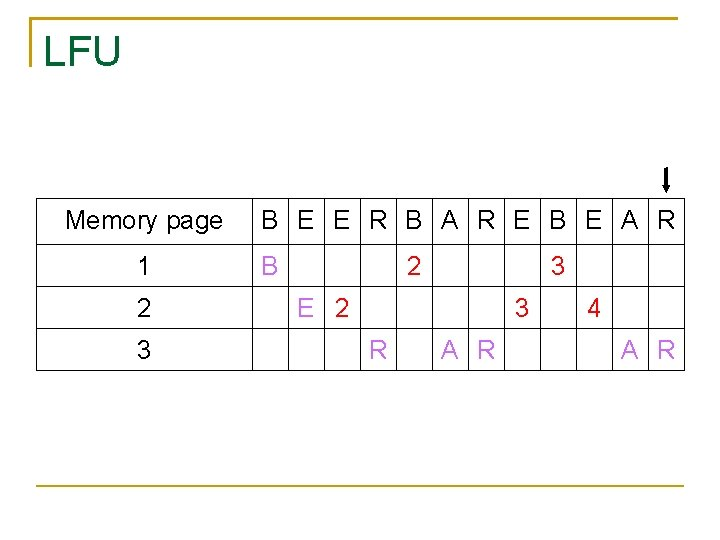LFU Memory page 1 2 3 B E E R B A R E B E A R B 2 3 E 2 3 R A R 4 A RLFU 7 page faults Memory page 1 2 3 B E E R B A R E B E A R B 2 3 E 2 3 R A R 4 A R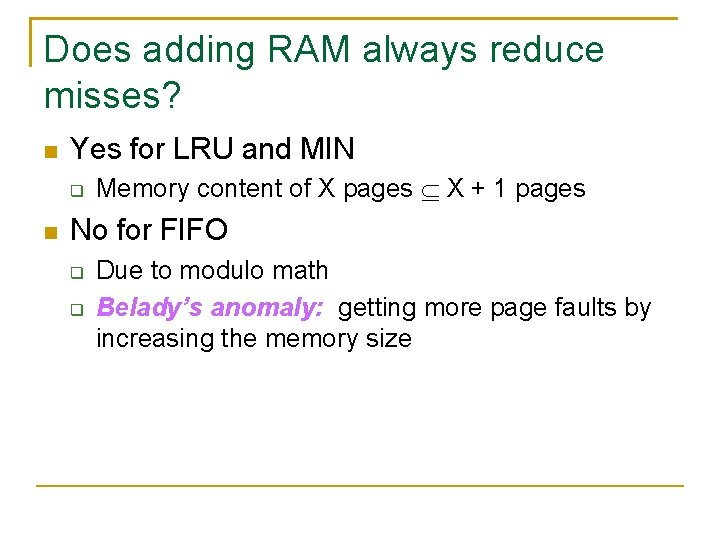Does adding RAM always reduce misses? Yes for LRU and MIN Memory content of X pages X + 1 pages No for FIFO Due to modulo math Belady’s anomaly: getting more page faults by increasing the memory size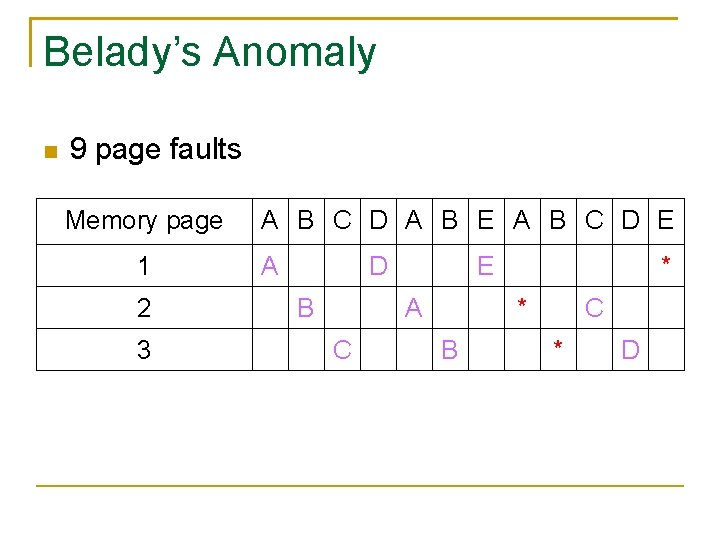Belady’s Anomaly 9 page faults Memory page 1 2 3 A B C D A B E A B C D E A D B E A C * * B C * DBelady’s Anomaly 10 page faults Memory page 1 2 3 4 A B C D A B E A B C D E A * B E * C D A E B D C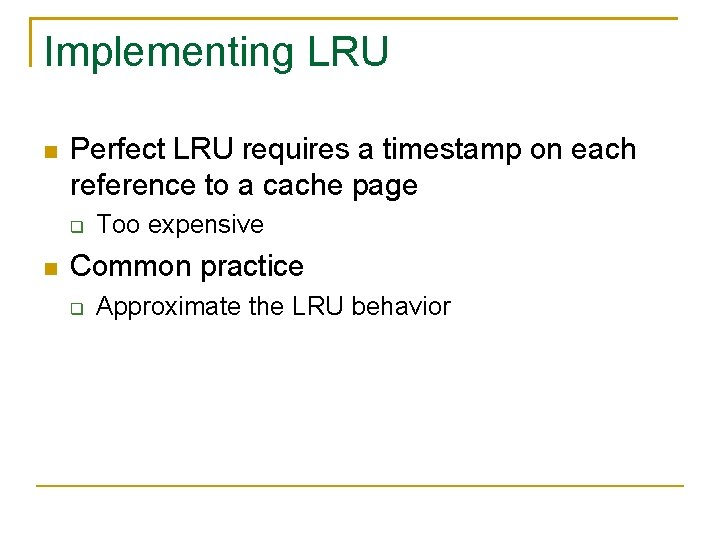Implementing LRU Perfect LRU requires a timestamp on each reference to a cache page Too expensive Common practice Approximate the LRU behavior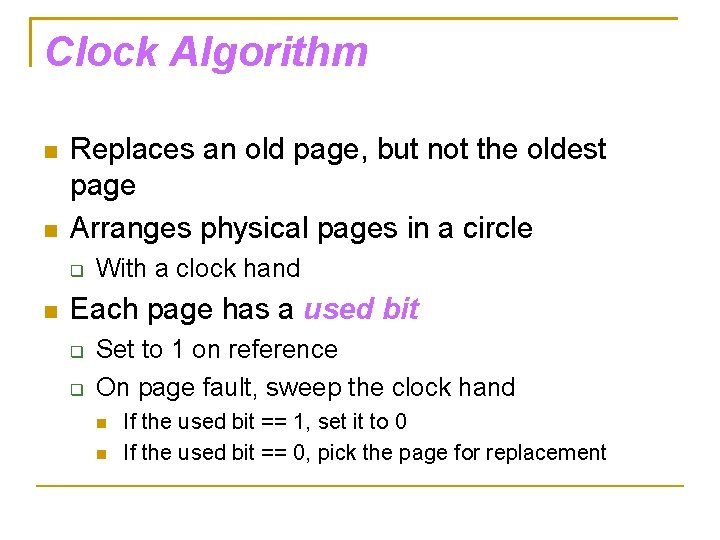Clock Algorithm Replaces an old page, but not the oldest page Arranges physical pages in a circle With a clock hand Each page has a used bit Set to 1 on reference On page fault, sweep the clock hand If the used bit == 1, set it to 0 If the used bit == 0, pick the page for replacementClock Algorithm 0 0 1 0 1 0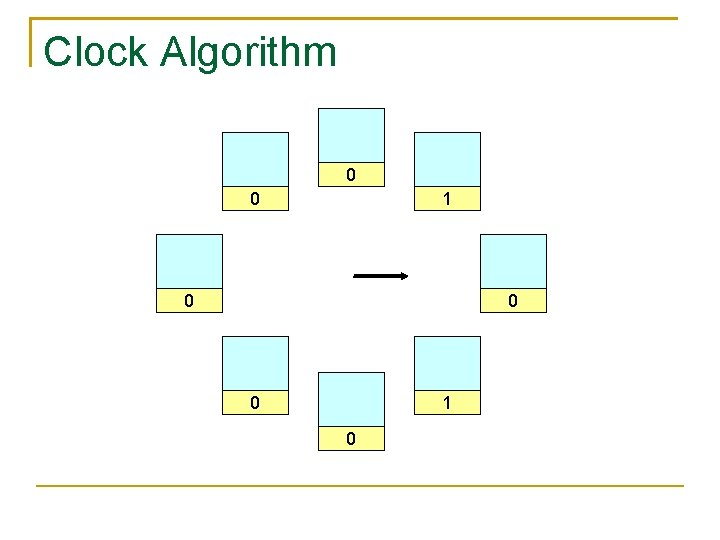Clock Algorithm 0 0 1 0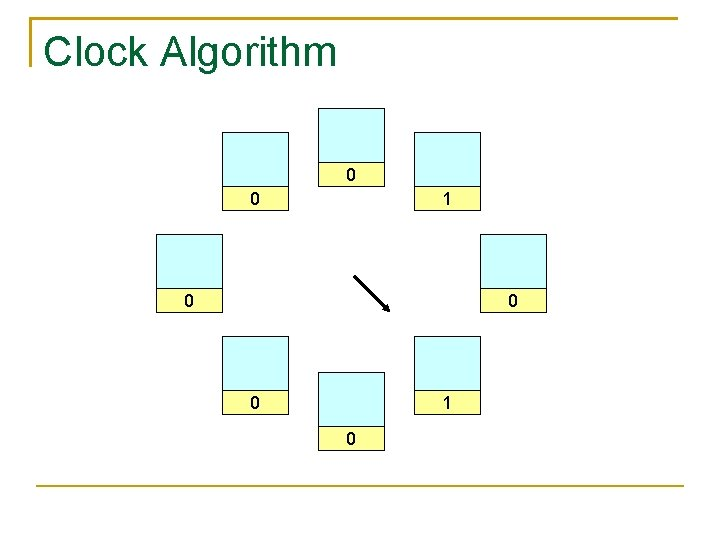Clock Algorithm 0 0 1 0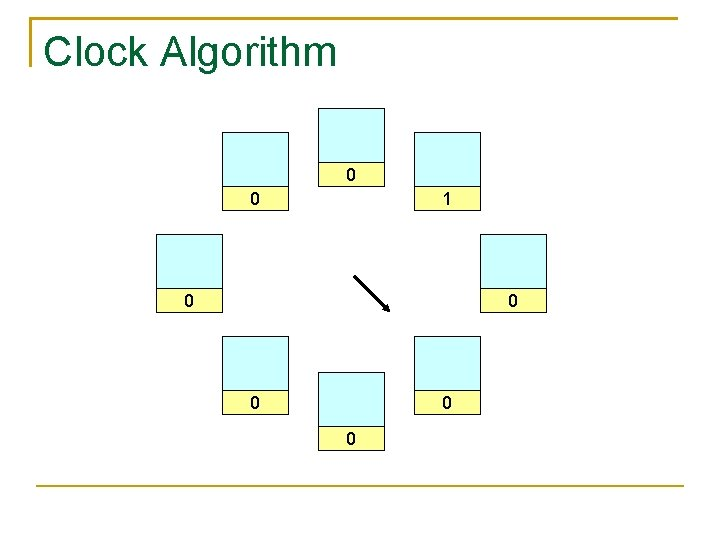Clock Algorithm 0 0 1 0 0 0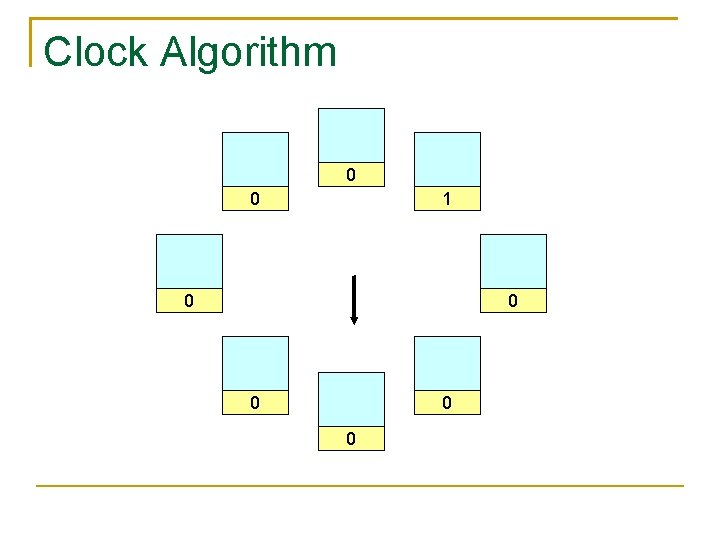Clock Algorithm 0 0 1 0 0 0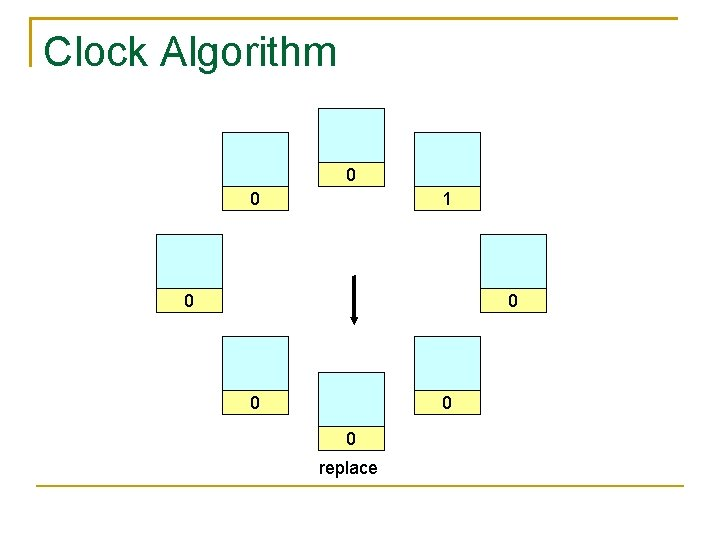Clock Algorithm 0 0 1 0 0 0 replaceClock Algorithm 0 0 1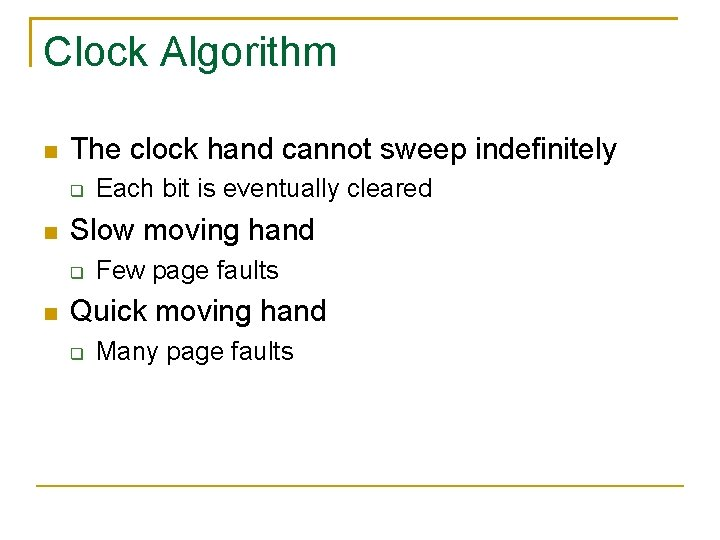Clock Algorithm The clock hand cannot sweep indefinitely Slow moving hand Each bit is eventually cleared Few page faults Quick moving hand Many page faultsNth Chance Algorithm A variant of clocking algorithm A page has to be swept N times before being replaced N , Nth Chance Algorithm LRU Common implementation N = 2 for modified pages N = 1 for unmodified pages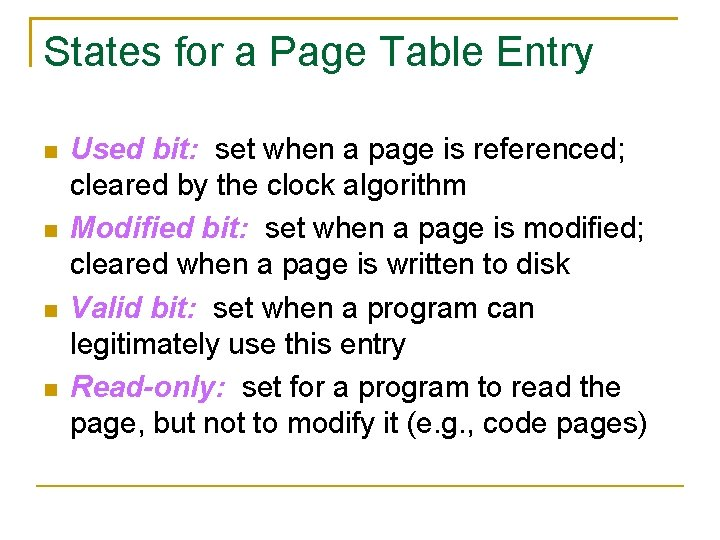States for a Page Table Entry Used bit: set when a page is referenced; cleared by the clock algorithm Modified bit: set when a page is modified; cleared when a page is written to disk Valid bit: set when a program can legitimately use this entry Read-only: set for a program to read the page, but not to modify it (e. g. , code pages)Thrashing Occurs when the memory is overcommitted Pages are still needed are tossed out Example A process needs 50 memory pages A machine has only 40 memory pages Need to constantly move pages between memory and disk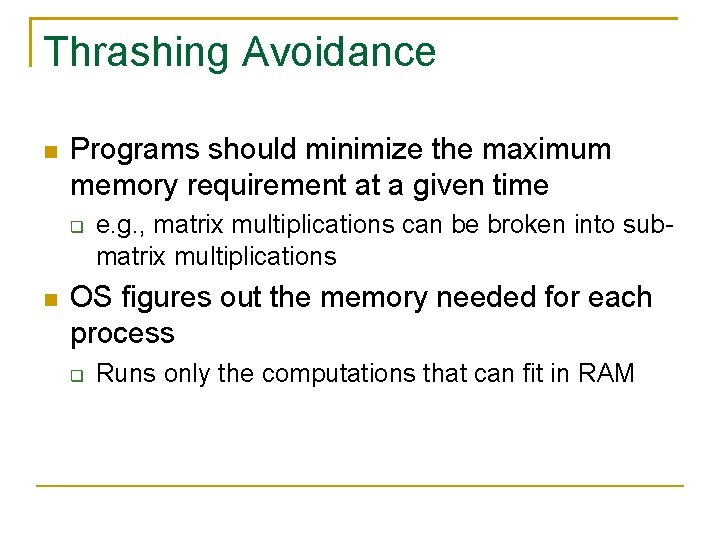Thrashing Avoidance Programs should minimize the maximum memory requirement at a given time e. g. , matrix multiplications can be broken into submatrix multiplications OS figures out the memory needed for each process Runs only the computations that can fit in RAM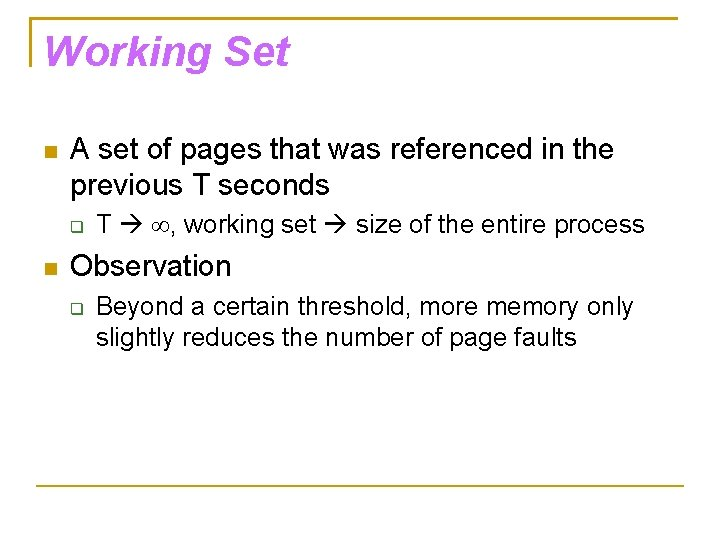Working Set A set of pages that was referenced in the previous T seconds T , working set size of the entire process Observation Beyond a certain threshold, more memory only slightly reduces the number of page faultsWorking Set LRU, 3 memory pages, 12 page faults Memory page 1 2 3 A B C D E F G H A D B C A C F D B G E H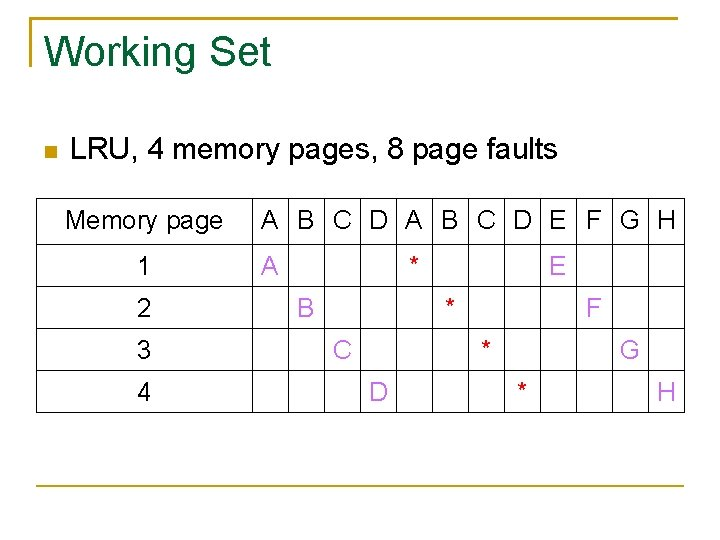Working Set LRU, 4 memory pages, 8 page faults Memory page 1 2 3 4 A B C D E F G H A * B E * C F * D G * H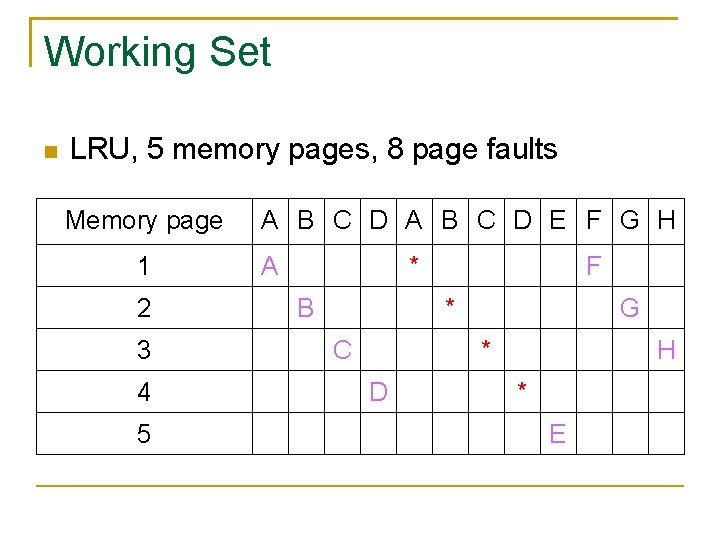Working Set LRU, 5 memory pages, 8 page faults Memory page 1 2 3 4 5 A B C D E F G H A * B F * C G * D H * E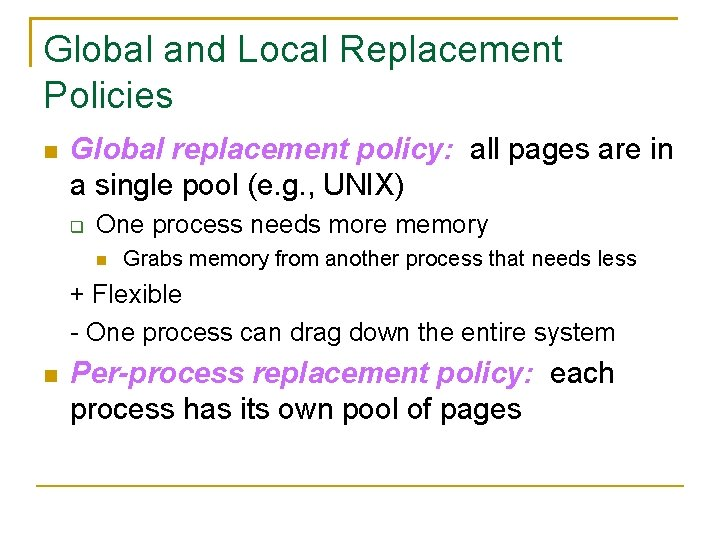Global and Local Replacement Policies Global replacement policy: all pages are in a single pool (e. g. , UNIX) One process needs more memory Grabs memory from another process that needs less + Flexible - One process can drag down the entire system Per-process replacement policy: each process has its own pool of pages